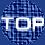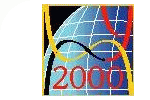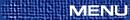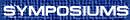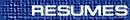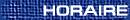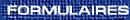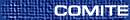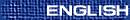Group Theory / Théorie des groupes
(Olga Kharlampovich, Organizer)

ROBERT BURNS, York
Constructible groups and a dichotomy in group laws

The class of ``constructible'' groups is obtained by closing the class of finite and soluble groups under the operations of forming extensions, subgroups, Cartesian products, and the ``local'' operator, i.e. inclusion of all groups which are locally among those already obtained. We characterize (almost) those group laws w = 1 such that every constructible group satisfying that law is nilpotent-by-locally finite of finite exponent. Thus semigroup laws and Engel laws represent special cases of such laws.

LISA CARBONE, Harvard University
Lattices on non-uniform trees

Let x be a locally finite tree. Then G = Aut(X) is a locally compact group. An `X-lattice' is a discrete subgroup L of G such that L\X has `finite volume'. We call L a cocompact lattice if L\X is finite, and a non-uniform lattice otherwise. If G is unimodular and G\X is finite, then Bass and Kulkarni ([BK]) have shown that G contains a cocompact X-lattice. It has been shown by the author ([C1]) that if G contains a cocompact lattice, is not discrete and acts minimally on X, then G also contains a non-uniform X-lattice. If we drop the assumption that G\X is finite, and assume only that G is unimodular, and that G\X is infinite but has `finite volume', then Bass and Lubotzky conjecture ([BL]) that G still contains a non-uniform X-lattice. We provide substantial evidence that this conjecture is true by proving it in a large number of cases ([C2]).

References

[BK ] H. Bass and R. Kulkarni, Uniform tree lattices. J. Amer. Math. Soc. (4) 3(1990).
[BL ] H. Bass A. Lubotzky, Tree lattices. Birkhauser, 2000, to appear.
[C1 ] L. Carbone, Non-uniform lattices on uniform trees. Mem. Amer. Math. Soc., 2000, to appear.
[C2 ]      , Lattices on non-uniform trees. 2000, in preparation.

JOHN DIXON, School of Mathematics and Statistics, Carleton University, Ottawa, Ontario  K1S 5B6
Using probabilistic methods to prove theorems in group theory

Since the 1960s there has been a series of results which state that certain events hold ``almost always'' in a group (or in a sequence of finite groups as the order tends to infinity). An early result of this type (conjectured by E. Netto around 1900), was proved by the speaker in 1969 : If two elements x, y are selected uniformly at random from the symmetric group Sn, then the probability that the alternating group An is contained in the subgroup áx,yñ tends to 1 as n® ¥. The object of this talk is to survey some of these results and to explain how they have been used recently to prove theorems in infinite groups which appear to have no ``probabilistic'' component. For example, it is shown in  how results of this type can be used to give an alternative proof of a theorem of T. S. Weigel [3-5]: A free group of rank n ³ 2 is residually S for any infinite set S of finite simple groups.

References

J. D. Dixon, The probability of generating the symmetric group. Math. Z. 110(1969), 199-205.

J. D. Dixon, L. Pyber, Á. Seress and S. Shalev, Residual properties of free groups and probabilistic methods. submitted for publication.

T. S. Weigel, Residual properties of free groups. J. Algebra 160(1993), 16-41.

, Residual properties of free groups II. Comm. Algebra 20(1992), 1395-1425.

, Residual properties of free groups III. Israel J. Math. 77(1992), 65-81.

ANTHONY M. GAGLIONE, US Naval Academy
Are some groups less discriminating than others

Here we show that the free nilpotent group of class c and rank r is not discriminating if min{c,r} is at least 2. We give the necessary preliminaries in order to understand the result.

STEVE GERSTEN, Utah, USA
To be announced

BOB GILMAN
Languages of free generators

It is well known that every subgroup of a finitely generated free group admits a set of Nielsen-reduced free generators with central significant factors. However, the situation changes if we require the set of generators to lie in a prescribed class of formal languages. We study the effect of this requirement and apply our results to a characterization of automatic groups.

SERGEI IVANOV, University of Illinois at Urbana-Champaign, Urbana, Illinois  61821, USA
Intersecting free subgroups in free products of groups

A subgroup H of a free product Õa Î I* Ga of groups Ga, a Î I, is called factor free if for every S Î Õa Î I* Ga and b Î I one has S H S-1 ÇGb = { 1} (by Kurosh's theorem on subgroups of free products, factor free subgroups are free). If K is a finitely generated free group, denote [`(r)](K) = max(r(K)-1,0), where r(K) is the rank of K.

It is proved that if H, K are finitely generated factor free subgroups of a free product Õa Î I* Ga then

 _r (H ÇK) £ 6 _r (H) _r (K)
and this estimate is sharp and cannot be improved. On the other hand, it is shown that if the factors Ga, a Î I, have no nontrivial elements of order less than or equal to q, where q £ 6, and H, K are finitely generated factor free subgroups of the free product Õa Î I* Ga then
 _r (H ÇK) £ 2 q+1q-1 _r (H) _r (K) .

In particular, if the factors Ga, a Î I, are torsion free groups then [`(r)](H ÇK) £ 2.8 [`(r)](H) [`(r)](K). It is also proved that if the factors Ga, a Î I, are linearly ordered groups then, as for free groups, one has

 _r (H ÇK) £ 2 _r (H) _r (K) .

OLGA KHARLAMPOVICH, McGill University
JSJ decomposition for finitely generated fully residually free groups

We will construct an algorithm to obtain the canonical JSJ decomposition for freely indecomposable finitely generated fully residually free groups. We also construct canonical embeddings of such groups into NTQ (nondegenerate triangular quasiquadratic) groups.

JOHN LABUTE, McGill University
On the cohomological dimension of one-relator pro-p-groups

Abstract: One of the central outstanding problems for pro-p-groups with one relator is the determination of their cohomological dimension. In particular, is such a group of cohomological dimension 1 or 2 if the group is torsion free? In our talk we will give a survey of known results concerning this problem.

ALEXEI MIASNIKOV, City College of CUNY
Exploiting links between the algebraic geometry over groups and logic

It turns out that the basic notions of the algebraic geometry over groups have some natural counterparts in the model theory and the universal algebra. On the one hand, this brings powerful methods of the model theory into the algebraic geometry and shows how to develop this type of geometry in a more general framework. On the other hand, these connections put new perspectives for some known methods in the universal algebra and lead to several new ideas in this area.

As an example, I will discuss new interesting links between the old Malcev's problem from the model theory and two recent problems in algebraic geometry which are due to B. Plotkin.

These are joint results with Vladimir Remesslennikov.

AKBAR RHEMTULLA, Alberta
Incompatible group orderings, local indicability and braids

Following a brief survey of results on which right orderable (RO) groups are locally indicable (LI), we go on to discuss which right orders on a subgroup H of of finite index in a RO-group G can be extended to right orders of G when G is not a LI-group. The results are applied to Artin Braid groups Bn, n ³ 5, which are known to be RO but not LI and the pure braids Pn which are of finite index in Bn and known to be twisted orderable.

LUIS RIBES, Carleton
Finite extensions of free pro-p groups

A description of virtually free pro-p groups having a free pro-p subgroup of index p is given in terms of free products. This extends a well-known result of Serre (torsion-free virtually free pro-p groups are free pro-p). It has also some consequences on the description of the fixed points of automorphisms of p-power order of free pro-p groups.

VLADIMIR SHPILRAIN, City College of CUNY
Measuring sets in finitely generated groups

To measure a finite (sub)set, one just counts elements of this (sub)set. To measure an infinite (sub)set, one can use different methods; one method that is becoming increasingly popular is considering intersections of the given infinite (sub)set with finite sets of ``uniformly'' increasing size, and then taking the limit of the size of such intersections. We use this method to measure various interesting subsets of the set of all elements of a given finitely generated groups. Based on these measurements, we address various probabilistic questions, like, for example: what is the probability for a randomly chosen element of a given group to be a generator? To belong to the commutator subgroup?, etc.

Answers to some of those questions turn out to be non-trivial, and sometimes even unexpected.

FRANCIS CHI-YU TANG, University of Waterloo
On the residual finiteness of the outer automorphism groups of certain generalized free products

It is a well-known result of G. Baumslag that automorphism groups of finitely generated residually finite groups are residually finite. Not much is known about the residual finiteness of outer automorphism groups of finitely generated residually finite groups. E. Grossman (1974) showed that the outer automorphism groups of surface groups are residually finite. Using recent results on conjugacy separability, we showed that the outer automorphisms of certain Seifert manifolds and generalized free products of free groups with cyclic amalgamation are residually finite.